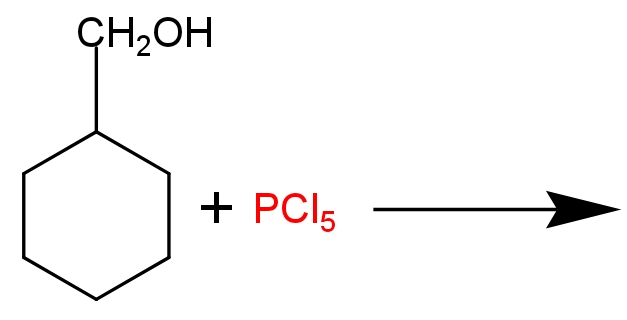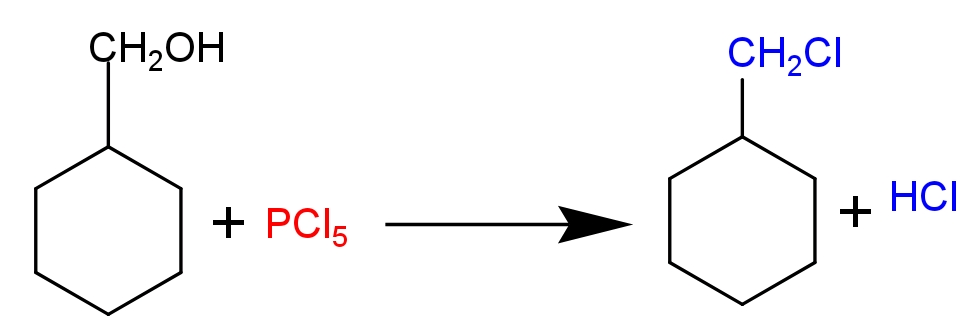# Draw the structure of major monohalo products in the following structure.Verified
175.2k+ views
Hint in a multi- product reaction the most likely formed product will be the major product of the reaction, which will be the structurally most stable form of product. Phosphorus pentachloride acts as a halogenating agent. It reacts with alcohols at room temperature and produces alkyl halide and fumes of hydrogen chloride. Phosphorus pentachloride found in solid state in room temperature.
Stronger bases are a poor leaving group.

Complete Step by step solution-
This reaction is an example of nucleophilic substitution reaction (${{\text{S}}_{\text{N}}}\text{2}$). In this reaction $\text{PC}{{\text{l}}_{\text{5}}}$ produces a nucleophile ($\text{C}{{\text{l}}^{\text{-}}}$ ion). It attacks alcoholic intermediates from the back side and substitutes the oxonium ion (electron deficient oxygen atom) and forms methyl cyclohexane chloride as a major product. Phosphoryl chloride ($\text{POC}{{\text{l}}_{\text{3}}}$) and $\text{HCl}$ is formed as a bi-product.In this reaction $\text{PC}{{\text{l}}_{\text{5}}}$ act as an electrophile, because$\text{PC}{{\text{l}}_{\text{5}}}$ attached with five highly electronegative chlorine atoms. Alcoholic group of benzyl chloride donate its lone pair to the phosphorus atom and forms oxonium ion by the release of $\text{C}{{\text{l}}^{\text{-}}}$ ion. Formation of oxonium ion makes it a good leaving group. $\text{C}{{\text{l}}^{\text{-}}}$ Ion attacks in electron deficient alkyl carbocation by ${{\text{S}}_{\text{N}}}\text{2}$ mechanism and forms benzyl chloride as a final product.
Note – ${{\text{S}}_{\text{N}}}\text{2}$Reaction takes place through an intermediate transition state and back side attack of nucleophile occurs from the less hindered side.
Primary alkyl group and non-polar solvent favour the ${{\text{S}}_{\text{N}}}\text{2}$ reaction.